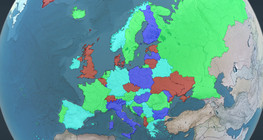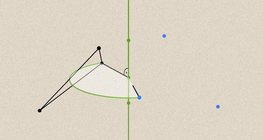Skaits: 0

Kopā: 0,00

0

# Surface area of spheres (demonstration)

### Surface area of spheres (demonstration)

The surface of a sphere consists of the set of points which are all at the same distance from a given point in space.

Matemātika

Atslēgvārdi

sfēras virsma, liels aplis, Matemātika, ģeometrija, virsma

Saistītie vienumi

### Saistītie vienumi#### Sphere

A sphere is the set of points which are all within the same distance from a given point in space.#### Four colour theorem

Colour a map with the fewest number of colours possible, so that no two adjacent regions have the same colour.#### Non-orientable surfaces

The Möbius strip and the Klein bottle are special two-dimensional surfaces with only one side.#### Perimeter, area, surface area and volume

This animation presents the formulas to calculate the perimeter and area of shapes as well as the surface area and volume of solids.#### Volume of spheres (Cavalieri´s principle)

Calculating the volume of a sphere is possible using an appropriate cylinder and cone.#### Volume of spheres (demonstration)

The sum of the volume of the ´tetrahedrons´ gives an approximation of the volume of the sphere.#### Geometric transformations – rotation

This animation demonstrates geometric rotation, a type of geometric transformation both in plane and space.#### Solids of revolution

Rotating a geometric shape around a line within its geometric plane as an axis results in a solid of revolution.